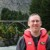# Can I Create an X-Y Matrix with Some Outputs Dependent from Other Outputs?

Six Sigma – iSixSigma Forums General Forums General Can I Create an X-Y Matrix with Some Outputs Dependent from Other Outputs?

Viewing 5 posts - 1 through 5 (of 5 total)
• Author
Posts
• #235464Abi
Participant

Good morning,

I’m quite new of the topic, I applied it just on some easy examples. Without entering in too detailed explainations, I have a process with a defined number of controllable inputs which generate the outputs.

The final aim of my project is to assign a score to my inputs in order to understand the more critical ones, which will be utilized to perform a design of experiment study.

I started creating a ‘Cause-Effect’ or ‘X-Y’ matrix of the process to study the severity of the inputs but I encountered a problem: some of my outputs depend from the inputs but also from other outputs. The relation for some output is Y=f(X,Y), for instance
Y1=f(X1,X2)
Y2=f(X1,X2,Y1)

Oversimplifying, it’s like having a pressurized container. My inputs are the temperature and external forces and my outputs are material stress and internal pressure. The material stress depends on the two inputs but also on internal pressure which is an output.

Does it exist an effective method or statistical tool to assign a score to the inputs?

Thanks

0
#235468Chris Seider
Participant

If output B is dependent on output A and output A is dependent on inputs 1, 2, and 3…wouldn’t output B just be dependent on inputs 1, 2, and 3 also?

1
#235477Abi
Participant

Yes of course I arrived to the same conclusion, and my idea was creating a scoring method in which I litterally count how many output each input affects, without making difference between direct or indirect influence, giving higher scores to the inputs which affect more outputs of the process.

My concern was that I don’t know if the relationships are linear or of lower or higher grade and since the influence can theoretically become very weak or stronger for this reason, I wasn’t sure it was the same.
For instance, literally inventing an example to explain my concern, if I have to score two input parameters X1 and X2, which generate two outputs Y1 and Y2.
If
Y1 = X2*X1^2
Y2 = X2*X1*Y1^2
it means both Y1 and Y2 are stronger dependent from X1 then from X2, then X1 would deserve an higher consideration than X2, but simply counting the output affected, they would be at the same level.
Of course I don’t have the precise relationships, otherwise the problem would be analitically solved and I would not need a scoring system.
I was looking for (if it exists, and if it’s applicable to my problem) a method which take into account that there are direct and undirect (function of a function) relationships.
I hope I explained my problem and my concerns. It is possible I’m creating my own problem and I’m totally wrong.

0
#235479Robert Butler
Participant

Just tallying how many times some X variable shows up in a series of regression equations (one for each Y response) isn’t going to address the importance of that X. If we use your example Y1 = X2*X1^2 and Y2 = X2*X1*Y1^2 then, in order for what you are saying to be true, the coefficients for the X’s in both of the models for Y1 and Y2 would have to be equivalent in magnitude – that is a unit change in X1 in Y1 would have the same effect as a unit change in X1 in Y2.

There is nothing that says this would be the case. Indeed, it is precisely because this is more often than not the situation that one can use the simultaneous equations for Y1 and Y2 to identify regions of “optimum” performance where “optimum” means the best trade off’s with respect to Y1 and Y2 given that both are dependent, to some degree, on the same variables.

I wouldn’t recommend trying to build a weighting scheme for your X’s. If you do have a situation where the number of X’s is such that you can’t build a screen design to check them then I would just bean count the number of times you think a particular variable is going to matter to the Y’s of interest, pareto chart the results, and go with the top N where N is the number of variables you can comfortably run in a screen design.

1
#235494Abi
Participant